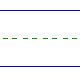Name: ______________________________________________ Date: _____________

Instructions: Find the sum.

 8 + 8 =9 + 5 =7 + 6 =8 + 3 =9 + 7 =6 + 7 =4 + 9 =6 + 6 =6 + 9 =7 + 5 =5 + 6 =6 + 8 =8 + 9 =8 + 5 =4 + 8 =8 + 7 =7 + 9 =9 + 9 =